### Home > A2C > Chapter 7 > Lesson 7.2.4 > Problem7-145

7-145.
1.This problem is a checkpoint for solving rational equations. It will be referred to as Checkpoint 13. Solve each of the following rational equations. Homework Help ✎

1.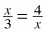2.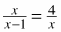3.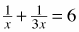4.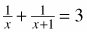5. Check your answers by referring to the Checkpoint 13 materials located at the back of your book.

If you needed help solving these equations correctly, then you need more practice solving rational equations. Review the Checkpoint 13 materials and try the practice problems. Also, consider getting help outside of class time. From this point on, you will be expected to solve equations such as these easily and accurately.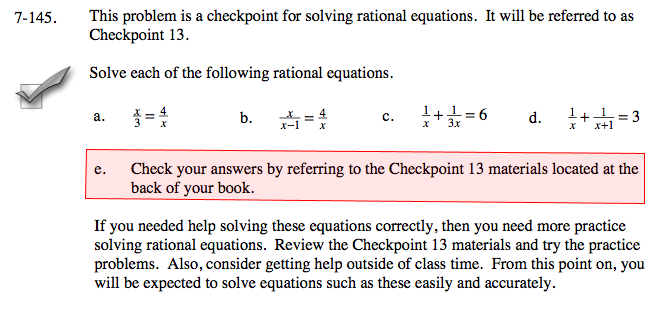Answers and extra practice are located in the back of your printed textbook or in the Reference Tab of your eBook.
If you have an eBook for A2C , login and then click the following link: Checkpoint 13 Answers and Practice